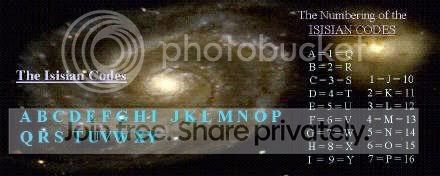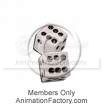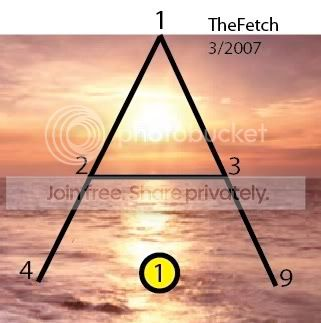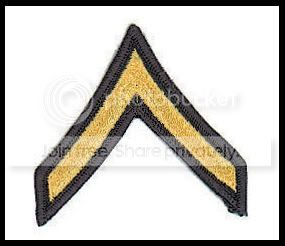## Upcoming Guests on Inside the Eye - Live!

Visit Inside the Eye - Live!, the new website for Inside the Eye - Live! radio show with The Fetch!

Show starts at 10:00am Eastern Call in or listen live!

Show Archives (including show summaries) from Apr 1, 2013. Previous show archives (without show summaries here).

Important note to visitors. Blogger has changed the HTML code used when this blog was originally created. What that means is that some pictures may not load, or pictures have disappeared from original linking URL's and are no longer available. Fixing any picture requires an entire reload of all pictures so that the pictures work with HTTPS, or Blogger will not allow any in story photo updates. Pages are currently being updated (freshened up). Be patient as pages get updated. Updates are based on various page use/visit priorities.

## Wednesday, September 5, 2007

### The Clock and the Circle

Here is a very fascinating code. I call it "the Clock and the Circle", wherein "the Circle" is inferred, then, to be, Pi. In order to properly effect this code, you need to be working with the right "substrate", meaning "representation" relative to the design.

Let's break down the numbers of time.

A day is divided into 24 hours. This is the Letter X. The 24 hour day is divided into 12 hour halfs, roughly equated to "night" and "day". The X can be divided in half as shown in the Da Vinci Code by Dan Brown. If you do not wish to accept Dan Brown's demonstration of the X being comprised of the inverted "V" as being male and the "V" as female, then consider this scan from "Starshift: 1948", a Masonic piece released in 1948.

In the above example, the X is being divided into two components. First, there are 4 "V's created off the emanation from the center point, and second, two "V's" as shown in The Da Vinci Code, representing Male and Female. In the case of the 4 V's eminating from the center point of the "X". The points were given "arbitrary" values of "6" such that the 4 "v's" times 6 = 24 = X Letter position. Nowhere did the author support "why" they would be "6's", even as he released the very code as to why indeed they should be counted as such. Second, the rationale provided by this author, a "Pickett", sets each of the V's in the vertical set (the upright and reverse V) as a value of Roman number "5", and so V as 5 plus V as 5 (reversed or mirrored on the upper V) arrives at the Roman value of ten.

It is not our position to provide "arbitrary values" to anything, as we hold that the design of the Alphabet is the key and a rational and logical analysis of this key reveals the Construct in a precise and predictable fashion. In the case of the "V's" having a "value" of "6", this is well established by the Isisian Codes, which as shown here reveals that V is located at number 6.In addition to the labials of F and V holding the position of the 6 column, the very phonetic of the word FIVE (FV) too is located at number 6. This core truth is key to understanding the entire Illuminatus system:

1+2+3+4+5=15=1+5=6

Hence the V as Female "5" is added from 1 to itself to reveal the 15th Letter of O, which is the Feminine "egg", "o-varies", "o-pen-is", etc. Five is 6.

The A as Six, The Secret of the Serpent Master Key

Here is another Key to the Illuminatus System, and indeed there are many "keys", but they are far different from "Keys". Note that the word KEY contains 2/3 of the word EYE. It is often necessary to have the "keys" before you can truly "see". This "key" is critical.

The Master Number is 11011. This is formed by the rhyming pattern of A, J, and K, wherein the A is placed at 1, the J is placed at 10, and the K is placed at 11. I recommend you write these out as you go along. Each of these numbers are "the Ordinal Values", or their regular placement in the Alphabet.

Turn now to Genesis, Pi (3:14):The LORD God said to the serpent, "Because you have done this, cursed are you above all cattle, and above all wild animals; upon your belly you shall go, and dust you shall eat all the days of your life. - Revised Standard Version

What this means is that whenever you have a "1"s showing, or "6's", it is oft necessary to consider the possibility that they may be either "1's" if 6's, or 6's if 1's. The reason for this is again in the summation tables of the Letters.1 = 1 = A
2 = 3 = B
3 = 6 = C
...
10 = 55 = J
11 = 66 = KWhen you have AJK, it has a declared value of 1,10,11, as a process of its ordinal letter placements. Set them up as 11011 as a single unit, "go the belly of the Serpent", aka, "snake eyes" when playing "craps", and you will arrive at the 6's on the belly, or what is resting on the table of the "crap table".

That "1's = 6's" from a "Kabballistics" sense can easily be inferred through studying the the Kabballah as practiced by Setian centric forces, which is the work of Rabbi Ginsberg at Inner.org. At this site is an article that seeks to deal with "Kabballah and String Theory",

"The full spelling of the letter yud is: yud (10), vav (6), dalet (4). The two additional letters, the vav (6) and the dalet (4) themselves equal the original yud (10). The full spelling of the yud is thus to be understood as an equation: 10 = 6 plus 4. The 10 dimensions of reality divide into two categories, one of 6 and the other of 4.

In Kabbalah, the vav (6) is considered the "male" element of reality whereas the dalet (4) is considered the "female" element. The "female" principle of reality is "revelation" whereas the "male" principle is concealed within the "female."

These are literally false conclusions as the Isisian Code System so readily reveals, and a subject that we will seek to deal with at a later time after more foundational material is provided to aid in the ascertainment of the esoteric codes in play, however, suffice it to say that Rabbi Ginsberg is using (essentially) the precise number patterns we are using relative to the X (10).

The "vav", 6, is considered a 1.

The 6 and the 4 (used in an additive formula 6+4=10) are being linked together to arrive at 10 (X), while Continental Mason systems attributed to the 4 V's as eminating from the center of the X to have values of 6 and hence 4*6=24=X Letter Placement. In addition, the "summative formulas" of letter placements from 1:X is too revealed as being "in play", i.e., C = 3 = 1+2+3 = 6 = 1 as C is the alignment of the Sun, Earth, and Moon in a perfect line to create an eclipse.

The "1's" as "6's"
It is critical to understand intuitively that nature of V's as "6's" and "1's" as "6's". What Dan Brown did not reveal when he inferred that X's are both male and female, that the rising (convex) chevron (reversed V) is male and the ascending (concave) chevron (V) is female, is precisely where the upper or rising chevron as an inverted V is precisely "the male".This is so because the "the male" is "Osiris" who "is the Sun" as it "arises" on the horizon. The Letter A is oft referred to as "the Pyramid Letter", "the One Eye'd God", etc. The number sequence as shown is taken from Plato's Timaeus,

After this he filled up the double intervals [i.e. between 1, 2, 4, 8] and the triple [i.e. between 1, 3, 9, 27] cutting off yet other portions from the mixture and placing them in the intervals, so that in each interval there were two kinds of means, the one exceeding and exceeded by equal parts of its extremes ----This entire compound he divided lengthways into two parts, which he joined to one another at the centre like the letter X, and bent them into a circular form, connecting them with themselves and each other at the point opposite to their original meeting-point; and, comprehending them in a uniform revolution upon the same axis, he made the one the outer and the other the inner circle. - source

There is a whole philosophy regarding Pi, the Letter A, and the Letter H, imbedded within those phrases, but for now, understand that the "1" is the tip of the A, yet it still maintains a hidden base (6) (2*3=6=3+2+1=C)Hence this is the "1" while the V is a "five" and 1+2+3+4+5 = V = the O letter is the Feminine, to arrive at the Letter Y as the child as a product of 3^2 + 4^2 = 5^, wherein the Letter Y is placed at 25, thus revealing the formula.

Solving for the Clock and the Circle

Now that you have a rational basis to understanding the relationship of V and inverted V to either being "5" or "6's", and that each could be said to have a value of 1, and that 1's are "6's", we can now solve for the "Clock and the Circle".

Study first this clock, and then the subsequent clock.

What is the difference? Notice how the upper clock on the left uses IIII to represent 4, while the clock on the bottom uses IV to represent 4?

Which one is right?

Why one or the other? Here are some observations.

Hickory Dickory Dock

Here is a tale that is perhaps too, a "key". Follow along.

Hickory dickory dock, the mouse ran up the clock
The clock struck one, the mouse ran down
Hickory dickory dock

it goes on till

Hickory dickory dock, five mice ran up the clock
The clock struck five, the mouse ran down
Hickory dickory dock

Now study the two clocks. If V's are 6's and 6's are 1's, how many "1's" are in the first clock? What is the pattern?

If V's are 6's and 6's are 1's, how many "1's" are in the second clock? What is the pattern?

The Key is "through 5", or the V.

I = 1
II = 2
III = 3
IIII = 4
V = 1

I = 1
II = 2
III = 3
IV = 2
V = 1

Which pattern appears more correct? This is really a basic "IQ" form of "pattern recognition test". Obviously, if the KEY is 5, and the patterns are as follows:

Pattern 1 = 1,2,3,4,1
Pattern 2 = 1,2,3,2,1

And the SONG speaks about "running up and down the clock", does 1,2,3,4,1 run up and down the clock or does 1,2,3,2,1 run up and down the clock?

Obviously, the latter "runs up and down the clock", so it would be necessary to utilize the second clock for our analysis.

So here is how it is done.

I = 1 = 6
V = 1 = 6
X = 11 = 66 = (2)

Now watch what happens to our "clock".

I = 1 = 6
II = 2 = 66
III = 3 = 666
IV = 2 = 66
V = 1 = 6
VI = 2 = 66
VII = 3 = 666
VIII = 4 = 6666
IX = 3 = 666
X = 2 = 66
XI = 3 = 666
XII = 4 = 6666

How many "6's" are there? 30 sixes.

6 times 30 = 180 degrees, or half the circle.
30 times 12 = 360 degrees, or the full circle.

Hence the Code reveals Pi in either conceptual 180 degrees of the division of day and night, or if we allow for the "30" to be multiplied by the 12 hours, we arrive at 360 degrees of the circle.

PS. So why would the IV be changed to a IIII? What would such reveal? Why, 32, of course. The decimal digits of Pi..

I bet you never saw this little ingenious code before.Anonymous said...

Im having a mouse synch and just thought I would eek my way into something else about the clock. The first one anyone, the second picture is not showing up! :P

If you count all the V's there are 12
If you count all the I's there are 20

Of course the hands of time go around these numbers twice. To indicate as you did, day and night.

Which is TL TL ...and considering the puns of it all, one of them is that 'start your way at the bottom and work your way up' which would reveal a TaLe/tail/tell/tell-tale:

T
H
G
I
L

Concentrated Energy

T
H
17
L

T
H
Q
L

T
QH
L

T
CUE H
L

T
CUE 8
L

LIGHT=56
but this? = 69

the 12 + 20 + 12 + 20 = 64

Little = Concentrated Energy

Love,
-sky-Anonymous said...

argh

'the first one anyone,' = the first one anyway,

eek eek

-sky-Anonymous said...

Or as Golden Dawn has it, "Zero equals one."

And Eisen says, "A BE C". A, as first term, IS (or = to) C, third term.

So if valid, including all the above, we get X. X for variable, according to precept or method.

Good sleuthing in any case.

The stave Gebo, X, means gift = energy exchange. From the gods to man... So this reflects all the above. And it's yet the same motif(s) over and over.

Anyway, Gebo is placed at 7th position. 1:7 = 28 = 10 = 1

So according to Golden Dawn we've a big fat 0. And I just realized that - tho this was meant to be a joke - this may actually refer to the concept of a Circuit.

There are 24 staves. So 28-24 = 4. Or maybe 24 - 1 = 23 = 5 or 6 - 1 = 5

5, the (F)V thing...

2 V's. Gebo.

This appears to be another box. Not just my gaming just above. It would be nice to see how this stuff applies to psyche or Anything to do with living in this corporatized mayhem. Hopefully my own right brain will figure this out. As it is now this is nice diversion but we've had a belly full of esoteric diversion for two decades now with very little to show.

Unknown said...

Please delete my raving just above. Meant to say that's quite the iffy biblical passage to assume a 6=1 transposition. Surely there are many others which might be projected upon or exploited. But it seems to have worked out. So I'll shut up. What can I find a copy of that cool masonic book about the letters? Or how can I get a Clearer image of your scan?

Unknown said...

The very last sentence confuses me. What do you mean by pi having 32 decimal digits? Pi technically has an infinite number of decimal places, and therefore cannot be written as a decimal.